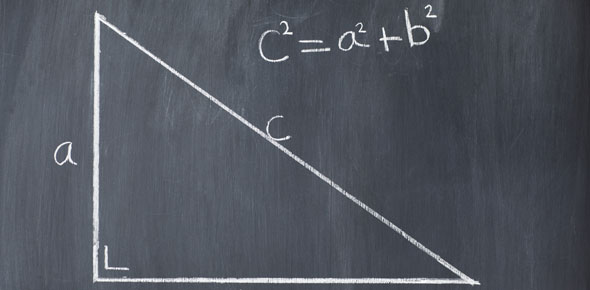# Solving Right Angled Triangles

5 Questions | Attempts: 647
ShareSettings• 1.
In a right angle triangle ABC, AB = 6 cm, AC = 10 cm and angle ABC = 90 degrees. The length of side BC is
• A.

11.7 cm

• B.

16 cm

• C.

8 cm

• D.

10 cm

• 2.
In a right angle triangle ABC, AB = 12 m, angle ABC = 90 degrees and angle BAC = 60 degrees. The length of side AC is
• A.

6 cm

• B.

24 cm

• C.

10.4 cm

• D.

16.5 cm

• 3.
In a right angle triangle ABC, AB = 12 cm,. BC = 10 cm and angle ABC = 90 degrees. The size of angle ACB is
• A.

39.8 degrees

• B.

56.4 degrees

• C.

33.6 degrees

• D.

50.2 degrees

• 4.
Point B is 5 m south of point A,  point  C is 8 m east of point B and point D is x m east of point C. If the distance from A to to D is 13 m then the value of x is
• A.

4 m

• B.

9 m

• C.

6m

• D.

8m

• 5.
One end of  a piece of wire 14 m long is attached to the top of a vertical pole and the other end is attached to the ground at an angle of 70 degrees to the ground.  What is the height of the pole?
• A.

4.8 m

• B.

14.9 cm

• C.

13.2 m

• D.

38.5 m

## Related TopicsBack to top
×

Wait!
Here's an interesting quiz for you.